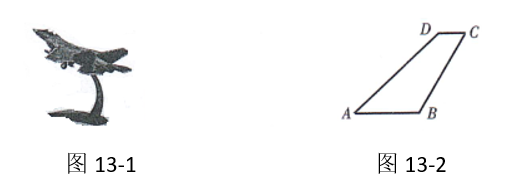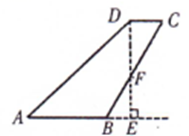【答案】\begin{aligned} & \because \mathrm{AB} / / \mathrm{CD}, \\ & \therefore \mathrm{DE} \perp \mathrm{CD} \\ & \therefore \angle \mathrm{FEB}=\angle \mathrm{FDC}=90^{\circ} \end{aligned}

\begin{aligned} & \therefore \angle C F D=30^{\circ}, C F=4, \quad D F=2 \sqrt{3} \\ & \because B C=8 \\ & \therefore B F=4 \\ & \therefore B F=C F \end{aligned}

\begin{aligned} \left\{\begin{aligned} \angle \mathrm{FEB} & =\angle \mathrm{FDC} \\ \angle \mathrm{CFD} & =\angle \mathrm{BFE} \\ \mathrm{BF} & =\mathrm{CF} \end{aligned}\right. \\ \therefore \triangle \mathrm{FEB} \cong \triangle \mathrm{FDC}(\mathrm{AAS}) . \end{aligned}

\begin{aligned} & \therefore \mathrm{BE}=\mathrm{CD}=2, \quad \mathrm{DF}=\mathrm{EF}=2 \sqrt{3} \\ & \because \angle \mathrm{D}=135^{\circ}, \quad \angle \mathrm{FDC}=90^{\circ} \\ & \therefore \angle \mathrm{ADE}=45^{\circ} \\ & \therefore \mathrm{AE}=\mathrm{DE}=4 \sqrt{3} \\ & \therefore \mathrm{S}_{\mathrm{ABCD}}=\mathrm{S}_{\triangle \mathrm{AED}}=\frac{1}{2} \mathrm{AE} \cdot \mathrm{DE}=\frac{1}{2} \times 4 \sqrt{3} \times 4 \sqrt{3}=24 \end{aligned}